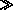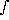# Saturation Vapor Pressure

Given T in Kelvin these equations will give es in milliBar.

from Fleagle and Businger, Vol.5, pg. 62 (QC880.F59)

The first law can be written as

L = T · (S2-S1) = U2 - U1 + Ps · (2 -1)

where11/1 and index 1 refers to the liquid phase and index 2 refers to the gas phase.

For an isothermal change of phase, the Clausius-Clapeyron equation has the form

d Ps/d T = L/[T · (2-1)]

Water vapor behaves like an ideal gas and21 for a change in state.

L2.5 · 103 Joules/gm

L2.824 · 103 Joules/gm over ice

Rw = R*/<mw>w = 8.3143/18.016 = 0.4615 Joules/gm/K

Ps =2 · Rw T

d Ps/d T = L/[T · (2-1)](L ·2)/T = (L · Ps) /(Rw · T2)

d log(es) = d Ps/Ps = (L/Rw) · (dT/T2)

loge(es) =(L/Rw) · (dT/T2) = -(L/Rw)|T->T0 + C = -L/(Rw · T0) + L/(Rw· T) + C

at triple point all 3 phases can exist in equilibrium, 0.0098° C and Ps = 6.11 mB

es(T=T0) = 6.11

L/Rw = 5417.12

L/(Rw T0) = 19.8313

6.11 · exp[ L/(Rw T0) ] = 2.504 · 109

es(T) = 6.11 · exp[ 5417( 1/T0 - 1/T) ] = 2.504 · 109 · exp[ 5417/T ]

Undocumented fit is used in the program watsat.F (over liquid)

es = 2.229 · 109 · exp[ -5385/T ]

Note this is the same equation as above, except that it assumes L = 2485.2 Joules/gm and T0 = 273.15 ° K

Another undocumented fit is given (but not used) in the program watsat.F

es = 0.001 · exp[ a/T + b + c log(T) + d · T + e · T2 ]

 coef over ice over water a -5631.1206 -2313.0338 b -8.363602 -164.03307 c 8.2312 38.053682 d -3.861449 · 10-2 -1.3844344 · 10-1 e 2.77494 · 10-5 7.4465367 · 10-5

From Rogers and Yau, pg. 16

es = 6.112 · exp[ a · (T-273.16)/(T-b) ]

 coef Rogers & Yauover water a 17.67 b 29.66

Murray, F.W. 1966. ``On the computation of Saturation Vapor Pressure'' J. Appl. Meteor. 6 p.204

es = 6.1078 · exp[ a · (T-273.16)/ (T-b) ]

 coef Murrayover ice Murrayover water a 21.8745584 17.2693882 b 7.66 35.86

Saucier, W.J. 1883. ``Principles of Meteorological Analysis'' Dover pg. 9 who uses values of Tetens (1930). Note, he used 10[(a'T)/(T-b')] with T in Centigrade so to convert into the form above a = log 10 · a' and b = 273.16-b'.

 coef over ice over water a 21.875 17.27 b 7.66 35.86